Courses

# RD Sharma Solutions - Ex-2.3, Fractions, Class 7, Math Class 7 Notes | EduRev

## Class 7: RD Sharma Solutions - Ex-2.3, Fractions, Class 7, Math Class 7 Notes | EduRev

The document RD Sharma Solutions - Ex-2.3, Fractions, Class 7, Math Class 7 Notes | EduRev is a part of the Class 7 Course RD Sharma Solutions for Class 7 Mathematics.
All you need of Class 7 at this link: Class 7

#### Question 1:

Find the reciprocal of each of the following fractions and classify them as proper, improper and whole numbers: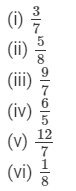Reciprocal of a non-zero fraction a/b is b/ a
(i)
Reciprocal of 3/7 is 7/3 It's improper fraction because numerator is greater than denominator

(ii)
Reciprocal of 5/8 is 8/5

It's improper fraction because numerator is greater than denominator

(iii)
Reciprocal of 9/7 is 7/9

It's proper fraction because numerator is less than denominator

(iv)
Reciprocal of 6/5 is 5/6

It's proper fraction because numerator is less than denominator

(v)
Reciprocal of 12/7 is 7/12

It's proper fraction because numerator is less than denominator

(vi)
Reciprocal of 1/8 is 8/1=8

It is a whole number

#### Question 2:

Divide: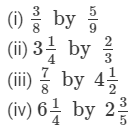####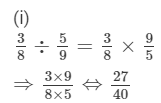(ii)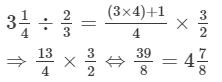(iii)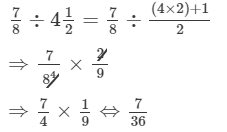(iv)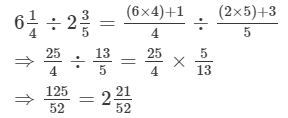#### Question 3:

Divide:

(i) 3/8 by 4
(ii) 9/16 by 6
(iii) 9 by 3/16
(iv) 10 by 100/3

(i)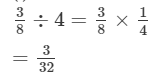(ii)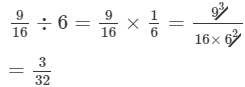(iii)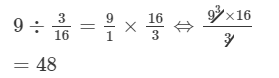(iv)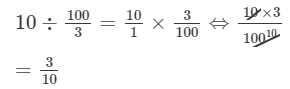#### Question 4:

Simplify: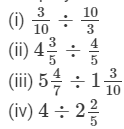(i)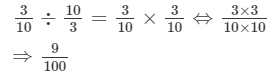(ii)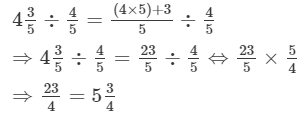(iii)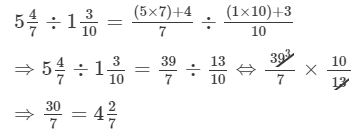(iv)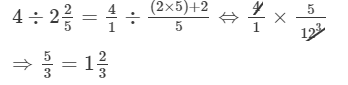#### Question 5:

A wire of length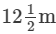is cut into 10 pieces of equal length. Find the length of each piece.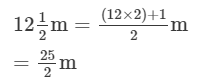Length of one piece =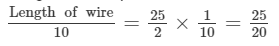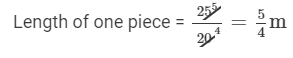#### Question 6:

The length of a rectangular plot of area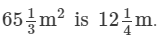What is the width of the plot?

Area of rectangle = Length of rectangle × Width of rectangle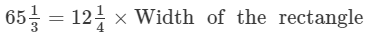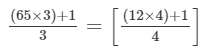×Width of the rectangle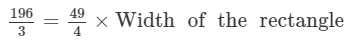Width of the rectangle =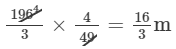#### Question 7:

By what number should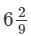be multiplied to get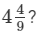Let the required number be x.

According to the question: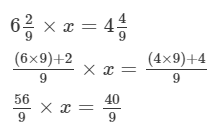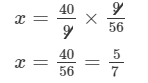#### Question 8:

The product of two numbers is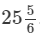If one of the numbers is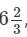find the other.

Let the required number be x.

According to the question: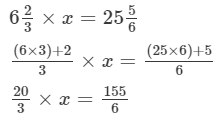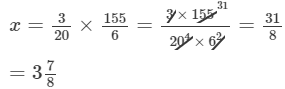#### Question 9:

The cost of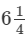kg of apples is Rs 400. At what rate per kg are the apples being sold?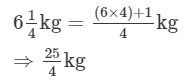Cost of  25/4 kg of apples = Rs. 400
Cost of 1 kg of apples =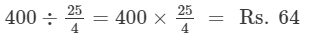#### Question 10:

By selling oranges at the rate of Rs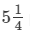per orange, a fruit-seller gets Rs 630. How many dozens of oranges does he sell?

Cost of 1 orange =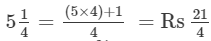Number of oranges sold =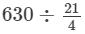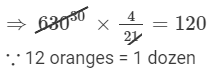#### Question 11:

In mid-day meal scheme 3/10 litre of milk is given to each student of a primary school. If 30 litres of milk is distributed every day in the school, how many students are there in the school?

Number of students in the school =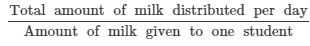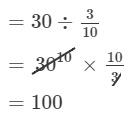#### Question 12:

In a charity show Rs 6496 were collected by selling some tickets. If the price of each ticket was Rs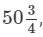how many tikets were sold?

Number of tickets sold =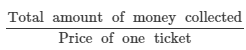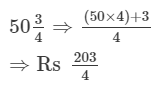Number of tickets sold =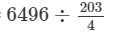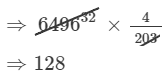The document RD Sharma Solutions - Ex-2.3, Fractions, Class 7, Math Class 7 Notes | EduRev is a part of the Class 7 Course RD Sharma Solutions for Class 7 Mathematics.
All you need of Class 7 at this link: Class 7Use Code STAYHOME200 and get INR 200 additional OFF Use Coupon Code
All Tests, Videos & Notes of Class 7: Class 7

### Top Courses for Class 7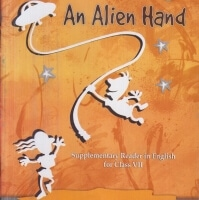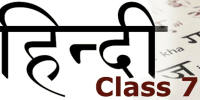## RD Sharma Solutions for Class 7 Mathematics

97 docs

### Top Courses for Class 7Track your progress, build streaks, highlight & save important lessons and more!

,

,

,

,

,

,

,

,

,

,

,

,

,

,

,

,

,

,

,

,

,

,

,

,

,

,

,

,

,

,

;